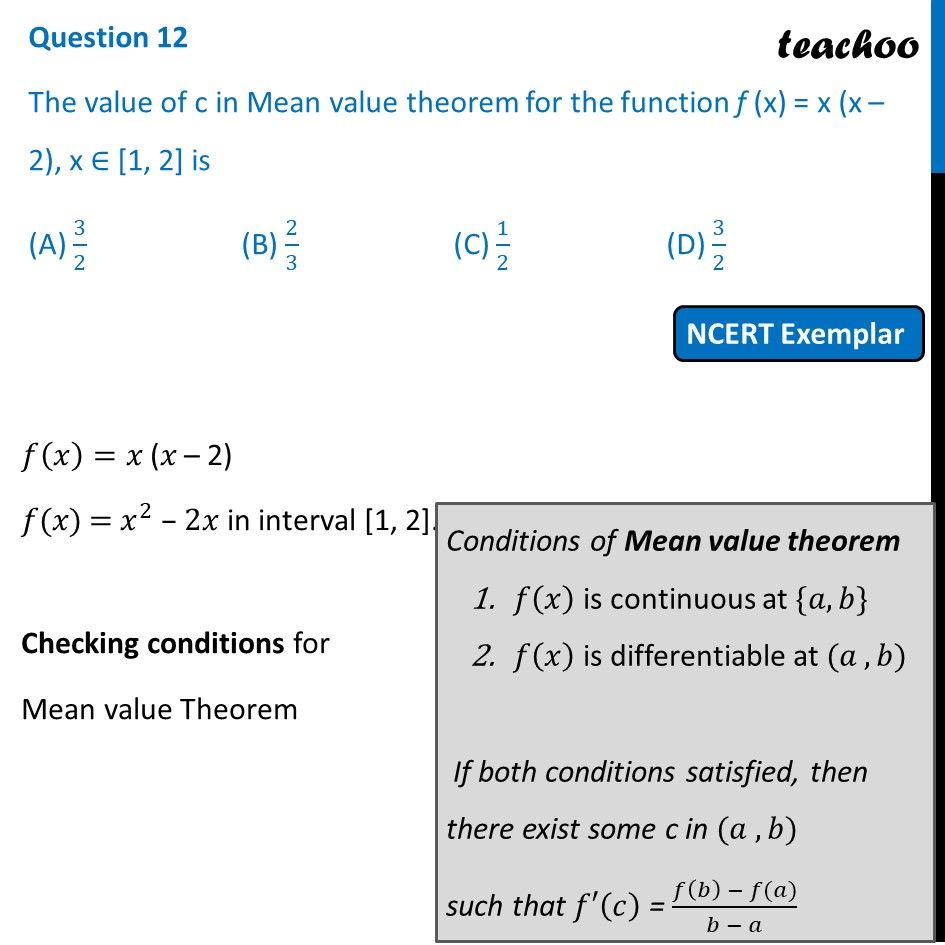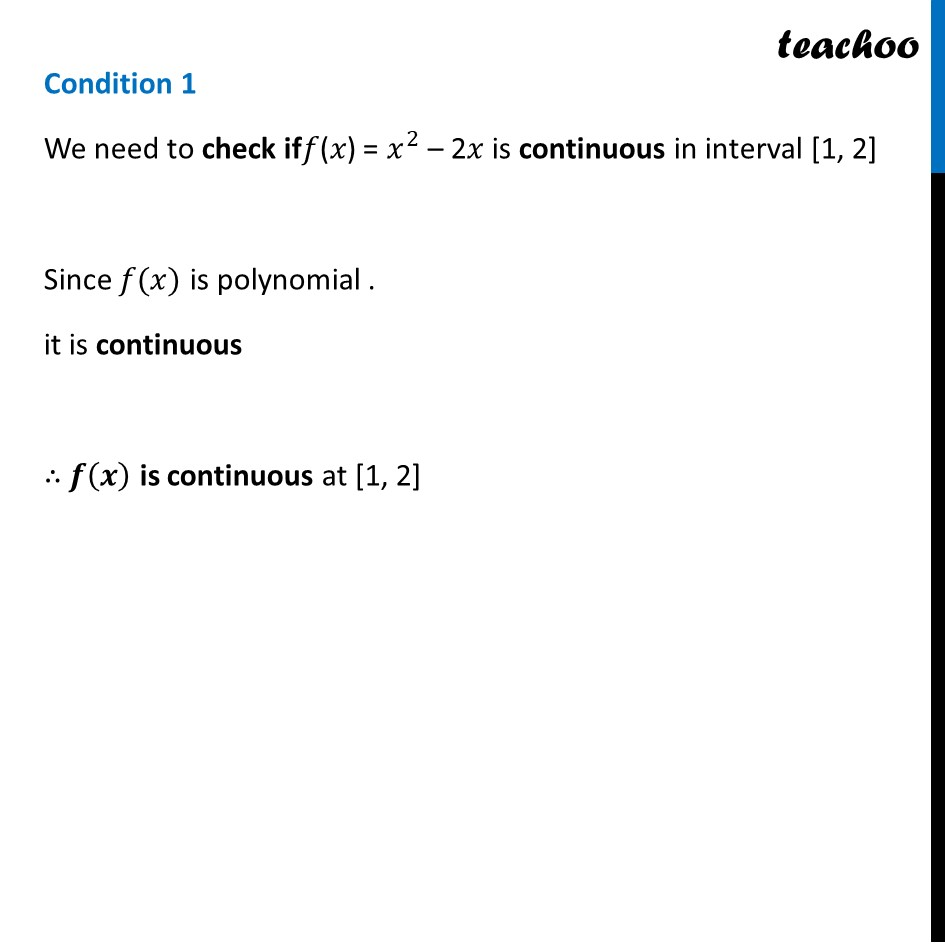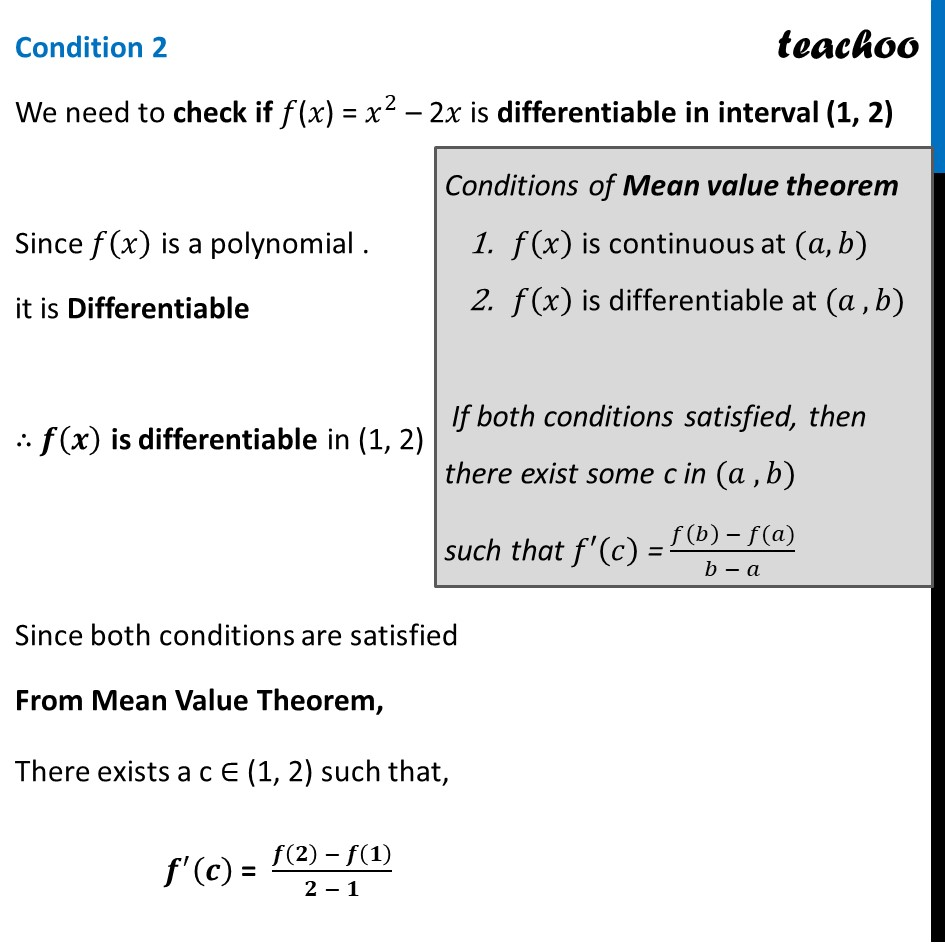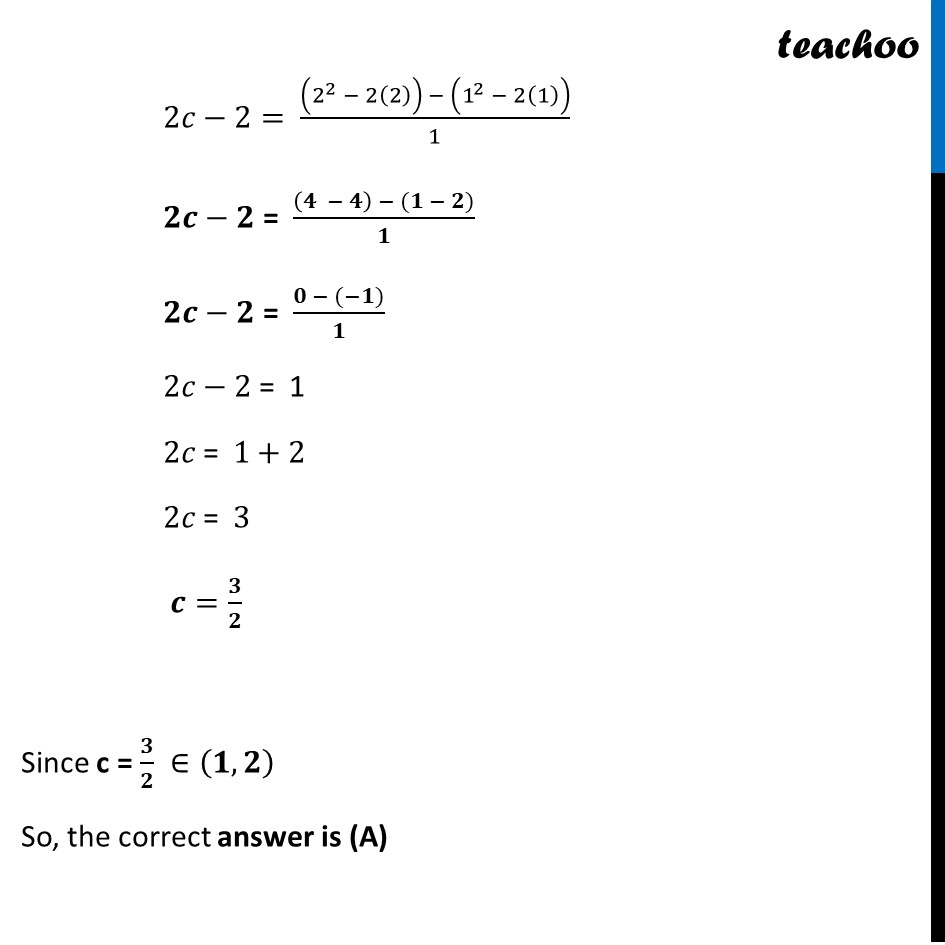NCERT Exemplar - MCQs

Chapter 5 Class 12 Continuity and Differentiability
Serial order wise

## (D) 3/2

This question is similar to Example 43 - Chapter 5 Class 12 - Continuity and DifferentiabilityGet live Maths 1-on-1 Classs - Class 6 to 12

### Transcript

Question 12 The value of c in Mean value theorem for the function f (x) = x (x – 2), x ∈ [1, 2] is (A) 3/2 (B) 2/3 (C) 1/2 (D) 3/2 𝑓(𝑥)=𝑥" (" 𝑥" – 2)" 𝑓(𝑥) = 𝑥^2 – 2𝑥 in interval [1, 2]. Checking conditions for Mean value Theorem Conditions of Mean value theorem 𝑓(𝑥) is continuous at {𝑎, 𝑏} 𝑓(𝑥) is differentiable at (𝑎 , 𝑏) If both conditions satisfied, then there exist some c in (𝑎 , 𝑏) such that 𝑓′(𝑐) = (𝑓(𝑏) − 𝑓(𝑎))/(𝑏 − 𝑎) Condition 1 We need to check if𝑓(𝑥) = 𝑥^2 – 2𝑥 is continuous in interval [1, 2] Since 𝑓(𝑥) is polynomial . it is continuous ∴ 𝒇(𝒙) is continuous at [1, 2] Condition 2 We need to check if 𝑓(𝑥) = 𝑥^2 – 2𝑥 is differentiable in interval (1, 2) Since 𝑓(𝑥) is a polynomial . it is Differentiable ∴ 𝒇(𝒙) is differentiable in (1, 2) Since both conditions are satisfied From Mean Value Theorem, There exists a c ∈ (1, 2) such that, 𝒇^′ (𝒄) = (𝒇(𝟐) − 𝒇(𝟏))/(𝟐 − 𝟏) 2𝑐−2= ((2^2 − 2(2)) − (1^2 − 2(1)))/1 𝟐𝒄−𝟐 = ((𝟒 − 𝟒) − (𝟏 − 𝟐))/𝟏 𝟐𝒄−𝟐 = (𝟎 − (−𝟏))/𝟏 2𝑐−2 = 1 2𝑐 = 1+2 2𝑐 = 3 𝒄=𝟑/𝟐 Since c = 𝟑/𝟐 ∈(𝟏, 𝟐) So, the correct answer is (A)